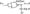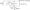# Analog and Mixed Mode VLSI Design June-July 2009

PART- A

1 a. State the reasons for the pedestal error, droop aperture error and sampling error.

b.State and explain specifications of ADC.

2  a. An 8 bit resistor string DAC was fabricated with a nominal resistor value of 1 kQ. If the process was able to provide matching of resistors to within 1%, find maximum INL and DNL of the converter. Assume = 5 V.

b. Explain generic (unweighted) current steering DAC and discuss the related mismatch errors.

c.   Design a 4 bit charge scaling DAC using a split array. Assume that – 5V and that C = 0.5 pF. Draw the equivalent circuit for D – 0001 and 0010 and determine the value of the output voltage.

3    a. Explain the principle of single slope ADC and the problems associated with it.

b. Draw the block diagram for 4 bit successive approximation ADC with = 5V. Explain the same. Trace the output at various stages for = 3.7V.

4    a. Explain the purpose of each stage of a voltage comparator. Also explain the working of 1st stage.

b. Show that multiplying quad acts as multiplier when all the MOSFETs in the multiplying quad have the same threshold voltage.

PART-B

5    a. Determine the ideal SNR of a 8 bit data converter with averaging of 20 outputs. (04 Marks)

b.  Draw the circuit arrangement used for decimation and averaging and explain the same. Determine the transfer function of the same.

c.   Bring out the principle of interpolation.

6    a. Describe CMOS process flow with neat sketches.

b.  Explain how MOSFET behaves as a capacitor. Also explain floating MOS capacitor.

7    a. Estimate the high-to-Iow and low-to-high delays in the circuits shown in figure Q7 (a).Fig. Q7 (a)

b.  Draw the arrangement for 4 bit pipelined adder and full adder bit implemented using dynamic logic.

c.   Explain the working of simple delay element using pass transistor and CMOS inverter.

8    a. Explain the limitations of inverter at the output of OPAMP, with the help of its transfer curve. How is it overcome?

b. Consider the AC small signal simplification of floating current source as in figure Q8 (b Assuming NMOS cascade output resistance is labeled Rncos, what is the small sign; resistance as seen by the test voltage Vtest?Fig Q8 (b)

c. Determine time constant of OPAMP with unity gain frequency of 100 MHz. Assume that al the outputs is fed back to the input. Also determine the settling time for 0.1% settling accuracy.